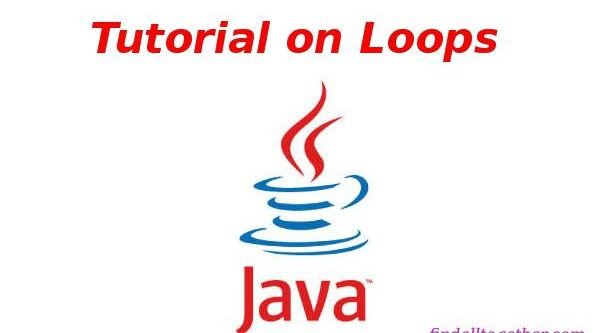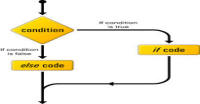# Loops in Java

In this article, we will study about loops in Java. Loops are the repetition of the code. For example, if I want to print 'harish' ten times then I will have to write Print command or 'harish' 10 times, but using loop I will write it only once and it will repeat to print 10 times.

Java has four types of loops:

1. while loop
2. do while loop
3. for loop
4. foreach loop

### while loop:

In this loop, we define a initial condition and a terminating condition. The loop starts with initial condition and ends at terminating condition. The syntax of while loop is :

while(condition){.........code.........}

This condition is same as condition of if else statement. While condition is true or non-zero this code will run again and again.

Look at this example:

```package flowkl;

public class Tutorials
{
public static void main(String args[])
{
int number = 0;
// while loop start
while(number != 10)
{
// code
System.out.println("harish");
// changing condition for terminate loop
number++;
}
}
}
```

In this code, number starts from 0 and the loop will run until number is less than 10. number is increasing 1 unit per repetition. This code will print 'harish' 10 times.

### do while loop:

This loop is also same as while loop but only difference is that it checks condition later and runs code first. In other words, it checks condition only for repetition. Its syntax is:

do{.....code.......} while (condition);

For example:

```package flowkl;

public class Tutorials
{
public static void main(String args[])
{
int number = 0;
do
{
System.out.println("harish");
number++;
}while(number != 10);
}
}
```

This code is also printing 'harish ' ten times. The different occurs only when I initialized number 10 or more value. In this case, while loop will not run but do while will. So, do while loop runs at least one time. Note the semicolon after while command in do while loop.

### for loop:

The syntax of for loop is:

for( initialize, condition, operation){ ....... code ....... }

Before discussing anything first take an example:

```package flowkl;

public class Tutorials
{
public static void main(String args[])
{
// example 1
//for loop with initialisation inside loop
for(int i = 0;i< 10;i++)
{
System.out.println("harish");
}

System.out.println("Initialization outside the loop");

// example 2
// Initialisation outside the loop
int j = 0;
for(;j<10;j++)
{
System.out.println("second type");
}

// example 3
// more than one condition
System.out.println("more than one condition");
for(int p = 1, x =5; p<10 && x < 30; p++, x*=2)
{
System.out.printf("p is %d and x is %d \n",p,x);
}

}
}
```

In first two types of loops, we see that first we have to initialize a variable, then we have to check a condition for loop and at last we have to change the value of variable (increment or decrement or something other). In for loop, we do all three things at same place. The for loop takes three arguments. Note these points:

1. initial value: This value is same as it is for first two types. In for loop, we can initialize a variable in two ways, one inside the loop (in brackets) and another before the loop. Note the syntax in above example. Each argument is separated by semicolon. If you are initializing value outside the for loop then also we have to put semicolon for initial value at initializing place (second example).
2. condition: condition is same as first two cases. It compares the initialized variable value and the given value. The loop will run until the condition is true.
3. operation: operation is also same as before. It changes the value of variable(s) after finishing code of loop each time. Operation may be any operation like addition, multiplication or even any complex operation.
4. In third example, note that we can define more than one variables, conditions and operations inside the loop by separating then with a comma.

#### Click on banner below to Learn: PHP with Laravel for beginners - Become a Master in LaravelHarish, a technical core team member at www.lyflink.com with five year experience in full stack web and mobile development, spends most of his time on coding, reading, analysing and curiously following businesses environments. He is a non-graduate alumni from IIT Roorkee, Computer Science and frequently writes on both technical and business topics.

### Related ArticlesPHP stands for PHP: Hypertext Preprocessor. PHP is known as a server side scripting and interpreter language for web ...
##### Introduction to PHPIn this tutorial, we will study about conditional statements (if else statements and switch statements). These statem...
##### Conditional statements in phpIn this tutorial, we will study about strings and operations upon strings. Due to long length of this section, I have...

### The Complete Web Developer Course - Build 25 Websites